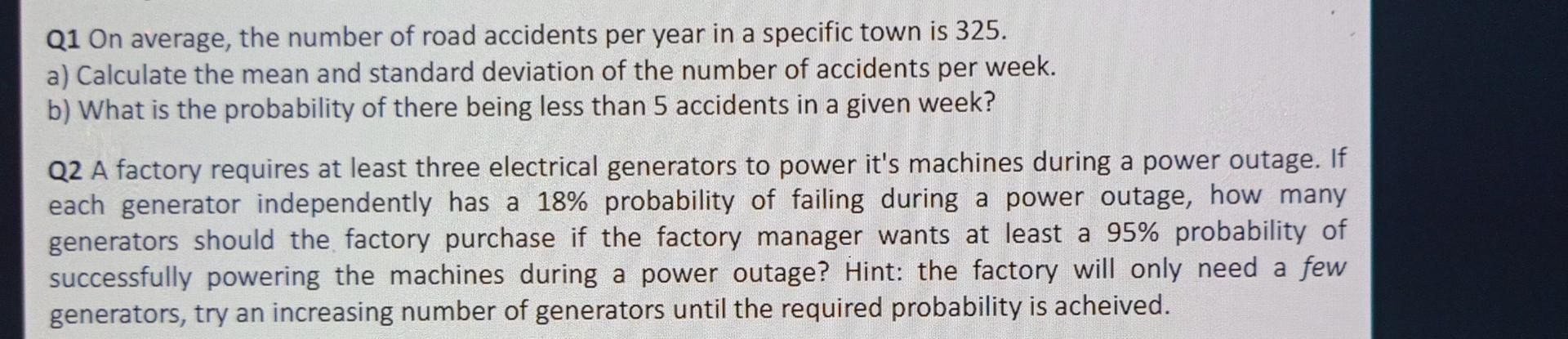# (Solved):   Q1 On average, the number of road accidents per year in a specific town is 325 . a) Calcul ...Q1 On average, the number of road accidents per year in a specific town is 325 . a) Calculate the mean and standard deviation of the number of accidents per week. b) What is the probability of there being less than 5 accidents in a given week? Q2 A factory requires at least three electrical generators to power it's machines during a power outage. If each generator independently has a \( 18 \% \) probability of failing during a power outage, how many generators should the factory purchase if the factory manager wants at least a \( 95 \% \) probability of successfully powering the machines during a power outage? Hint: the factory will only need a few generators, try an increasing number of generators until the required probability is acheived.

We have an Answer from Expert